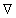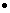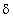## 10.3 The Divergence Theorem

The divergence theorem is the form of the fundamental theorem of calculus that applies when we integrate the divergence of a vector v over a region R of space. As in the case of Green's or Stokes' theorem, applying the one dimensional theorem expels one of the three variables of integration to the boundaries, and the result is a surface integral over the boundary of R, which is directed normally away from R.
The one dimensional fundamental theorem in effect converts thev in the integrand to an nv on the boundary, where n is the outward directed unit vector normal to it.
Another way to say the same thing is: the flux integral of v over a bounding surface is theĀ integral of its divergence over the interior.

Divergence Theorem: For R a reasonably nice region in whichv exists, we havewhere the normal is taken to face out of R everywhere on its boundary,R.

Proof